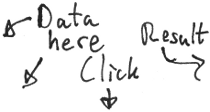# Bayesian Estimation Supersedes the t-test (BEST) - online

This page implements an online version of John Kruschke's Bayesian estimation supersedes the t-test (BEST), a Bayesian model that can be used where you classically would use a two-sample t-test. BEST estimates the difference in means between two groups and yields a probability distribution over the difference. From this distribution we can take the mean credible value as our best guess of the actual difference and the 95% Highest Density Interval (HDI) as the range were the actual difference is with 95% credibility. It can also be useful to look at how credible it is that the difference between the two groups is < 0 or > 0.

To try it out just enter some data below or run with the data that is already entered, the heights in m of the winning team of the 2012 NBA finals (group 1) and the winning team of Stanley cup 2012 (group 2). Data can be entered in almost any way, separated by spaces, commas, newlines, etc.

The MCMC method used is an adaptive Metropolis-within-Gibbs sampler described by Roberts and Rosenthal (2009). Everything is implemented in javascript and runs in the browser. If the output looks strange try to increase the number of burn-in steps and the number of sample steps.

Log
Data group 1
Data group 2Nbr of burn-in samples
Nbr of samples
Trace Plot - Difference of Means
Should look like a "hairy caterpillar"...
Distribution - Difference of Means
If the 95% Highest Density Interval does not include zero there is a credible difference!

## More Results - The Rest of the Parameters!

Even though the difference between the means of the groups usually is the main interest, BEST also estimates other parameters. Except for the means and SDs of the groups BEST estimates a measure of to what degree there are outliers in the data that makes the distribution of the data deviate from normality. This measure is labeled "Normality" below where a normality estimate < 1.5 indicates that the data isn't normally distributed. BEST is however robust to outliers to some degree while outliers are a problem for a classical t-test. More about the assumptions of BEST and the advantages of Bayesian estimation is found in Kruschke (2012).

Trace plot - Mean Group 1
Density - Mean Group 1
Trace plot - SD Group 1
Density - SD Group 1
Trace plot - Mean Group 2
Density - Mean Group 2
Trace plot - SD Group 2
Density - SD Group 2
Trace plot - Normality
Density - Normality
Trace plot - Difference of SDs
Density -Difference of SDs
Trace plot - Effect Size
Density - Effect Size
(μ₁ - μ₂) / sqrt( (σ₁² + σ₂²) / 2)
A Word of Caution. Even though this online version of BEST should give the same result as the method described by Kruschke (2012) I don't guarantee that it does. Use the version freely available on his site. If you want to know more about Bayesian statistics do check out his book, which is great, or some of the many other good introductory texts.
About. This page was made for fun by me, Rasmus Bååth, a PHD student at Lund University Cognitive Science, Sweden. Libraries used: jStat for probability distributions, Flot for plotting and JQuery for this and that. For css styling I used the Square Grid framework. If you have any suggestions for improvements feel free to drop me a message.